[中文] Search Internet Search HelloDBA
Oracle Technology Site. email: fuyuncat@gmail.com  MSN: fuyuncat@hotmail.com# Oracle 12c TOP－N Frequency Histogram

[中文]

Author:  fuyuncat

Source:  www.HelloDBA.com

Date:  2013-08-21 23:33:16

Share to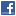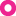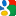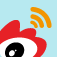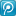In 12c, a new feature of the optimizer is that it provides new type histogram statistics data for the columns, TOP-N frequency histogram and HYBRID histogram. With them, the optimizer can choose a best execution plan more efficiently and accurately. Here I will talk about the top-n frequency, how the statistics gather process generate it, and how it impact the optimizer to calculate the selectivity.  <<<<<<<<<<<<<<<<<<<<<<<<<<<>>>>>>>>>>>>>>>>>>>>>>>>>>>>>>>>>>>>> A top frequency histogram is a variation on a frequency histogram that ignores unpopular values that are statistically insignificant. For example, if a pile of 1000 coins contains only a single penny, then you can ignore the penny when sorting the coins into buckets. A top frequency histogram can produce a better histogram for highly popular values. <<<<<<<<<<<<<<<<<<<<<<<<<<<>>>>>>>>>>>>>>>>>>>>>>>>>>>>>>>>>>>>> How to generate a top-n frequency histogram One thing we first need to know is that if you specify a non-default percentage, the behaviour of statistics gathering process will be similar to 11g. Therefore, to generate the new type of histograms in 12c, a necessary condition is that you should leave the estimate percent as default. for example. SQL代码 HelloDBA.COM> exec dbms_stats.gather_table_stats(ownname=>'OE',tabname=>'T_NTOP',method_opt =>'for columns A size 25');   According to the description of top-n frequency histogram, the histogram bucket number definitely smaller than the distinct value number of the column. Hence, another necessary condition is that the specified bucket size or the default bucket size preference setting (default is 254) is smaller than the distinct value number of the column. The statistics gathering process will first compute the approximate of distinct value number before further gather staistics data for the column. This step will involve SQL Analyzer to analyze a specified SQL according to the input parameter. The SQL will be like below, SQL代码 select /*+  full(t)    no_parallel(t) no_parallel_index(t) dbms_stats cursor_sharing_exact use_weak_name_resl dynamic_sampling(0) no_monitoring xmlindex_sel_idx_tbl no_substrb_pad  */to_char(count("A")),to_char(substrb(dump(min("A"),16,0,64),1,240)),to_char(substrb(dump(max("A"),16,0,64),1,240)),to_char(count("B")),count(rowidtochar(rowid)) from "OE"."T_NTOP" t  /* TOPN,NIL,NIL,ACL,RWID,U25,UU*/   The SQL Analyzer will not only get the result of this query, but also get other information according the options ( TOPN,NIL,NIL,ACL,RWID,U25,UU) by calling internal function during executing and analyzing this query. One of them is the top-n distinct values and their row number. While, if total row number of top-n values is less than the threshold (1-1/MNB) (Maximum Number of Buckets, one of important factor to determine choose frequency or height-balanced histogram, refer to http://www.hellodba.com/reader.php?ID=19&lang=EN) of not-null-value number of this column, the SQL Analyzer will discard the discard those top-n values, that is to say, the third necessary condition is that total row number of top-n values should be more than (1-1/MNB) of not-null-value number of this column. Because the smallest and largest distinct value will finally be involved into the histogram, the process will check if it's necessary to remove some value(s) from top-n values to clear vacancy for the smallest and largest distinct value. Those to be removed values would be the removable values. Finally, the percent of non-removable top-n distinct value row number (total row number of top-n values - removable row number of top-n values) in the not-null-value number of this column will determine the top-n frequency histogram could be used or not. That is, if the percent larger than the threshold (1 - (1/MNB)), it would generate a top-n frequency histogram. Note: If the smallest or largest distinct value is not included in the top-n distinct values so far, they will be involved into the histogram and replace the distinct values who have least row number. And the row number will be assigned as 1 since it's not gathered when compute the approximate data. In conclusion, to generate a top-n histogram, below conditions should be fulfilled, ** The estimate percent should be default; ** The histogram buckets fewer than distinct values; ** (total row number of top-n values - removable row number of top-n values)/(not-null-value number of the column) > (1 - (1/MNB)) DEMO I have an example to demonstrate TOP-N frequency generation. SQL代码 HelloDBA.COM> create table oe.t_ntop (a number, b number);      Table created.      HelloDBA.COM> begin     2    for r in (select level lv from dual connect by level<=30) loop     3      insert into oe.t_ntop select r.lv, level from dual connect by level<=r.lv*10;     4    end loop;     5    commit;     6  end;     7  /      PL/SQL procedure successfully completed.      HelloDBA.COM> exec dbms_stats.gather_table_stats(ownname=>'OE',tabname=>'T_NTOP',method_opt =>'for columns A size 25');      PL/SQL procedure successfully completed.      HelloDBA.COM> select histogram from all_tab_columns where owner='OE' and table_name='T_NTOP' and column_name='A';      HISTOGRAM   ---------------   HYBRID   Although I assigned bucket size to 25 which is less than distinct value number, 30, and used default value for the estimate percent, the process still failed to get a top-N frequency histogram. That could mean it failed to fulfil the last condition. Let's check the total row number of top-n values and the not-null-value number of the column as well as the threshold, SQL代码 HelloDBA.COM> select total_topn, total_notnull, round(total_topn/total_notnull*100,2) pct, round((1-1/25)*100,2) threshold from (select sum(case when rownum<=25 then cnt else 0 end) total_topn, sum(cnt) total_notnull, count(1) dvn from (select a, count(1) cnt from oe.t_ntop where a is not null group by a order by count(1) desc));      TOTAL_TOPN TOTAL_NOTNULL        PCT  THRESHOLD   ---------- ------------- ---------- ----------         4500          4650      96.77         96   The percent of (total row number of top-n values / the not-null-value number of the column) is larger than the threshold, that might mean there is removal row number of top-n values. SQL代码 HelloDBA.COM> select * from (select a, count(1) cnt from oe.t_ntop where a is not null group by a order by count(1) desc) where rownum<=25;               A        CNT   ---------- ----------           30        300   ... ...           6         60      25 rows selected.      HelloDBA.COM> select max(a) maxa, min(a) mina from oe.t_ntop;            MAXA       MINA   ---------- ----------           30          1   The smallest distinct value of the top-n values is not the smallest value of this column, so, the row number (60) of the smallest distinct value (6) of the top-n values is removable. Recalculate percent will be, SQL代码 HelloDBA.COM> select round((4500-60+1)/4650*100,2) adjusted_pct from dual;      ADJUSTED_PCT   ------------          95.51   It's smaller than the threshold (96), therefore, this column can not generate a top-n histogram based on the bucket size setting. Selectivity based on top-n frequency histogram. The calculation of selectivity based on top-n frequency histogram is not complex.   * If the predication value is one of the top-n frequency distinct value, the optimizer will use the bucket endpoint number of this value and sample size to calculate the selectivity.   * If the predication value is not one of the top-n frequency distinct value, the optimizer will the number of distinct value out of top-n values and their total row number to calculate the selectivity. For example, SQL代码 HelloDBA.COM> exec dbms_stats.gather_table_stats(ownname=>'OE',tabname=>'T_NTOP',method_opt =>'for columns A size 26');      PL/SQL procedure successfully completed.      HelloDBA.COM> select sample_size, density, histogram from all_tab_columns where owner='OE' and table_name='T_NTOP' and column_name='A';      SAMPLE_SIZE    DENSITY HISTOGRAM   ----------- ---------- ---------------          4650 .000107527 TOP-FREQUENCY      HelloDBA.COM> select endpoint_value, endpoint_number from dba_histograms where owner='OE' and table_name='T_NTOP' and column_name='A';      ENDPOINT_VALUE ENDPOINT_NUMBER   -------------- ---------------                1               1                6              61   ... ...               19            1751               20            1951   ... ...               30            4501   Notes: Because the smallest distinct value (1) is not included into the top-n frequency values when compute the approximate data, it replaced the value (5) with least row number. SQL代码 HelloDBA.COM> set autot trace exp   HelloDBA.COM> select * from oe.t_ntop where a = 20;      Execution Plan   ----------------------------------------------------------   Plan hash value: 1181773393      ----------------------------------------------------------------------------   | Id  | Operation         | Name   | Rows  | Bytes | Cost (%CPU)| Time     |   ----------------------------------------------------------------------------   |   0 | SELECT STATEMENT  |        |   200 |  1200 |     5   (0)| 00:00:01 |   |*  1 |  TABLE ACCESS FULL| T_NTOP |   200 |  1200 |     5   (0)| 00:00:01 |   ----------------------------------------------------------------------------      Predicate Information (identified by operation id):   ---------------------------------------------------         1 - filter("A"=20)   The data number of 20 in the histogram is 200(1951-1751), the calculated selectivity will be 200/sample_size=200/4650, therefore, the filtered row number is cardinality*selectivity=4650 SQL代码 HelloDBA.COM> select * from oe.t_ntop where a = 2;      Execution Plan   ----------------------------------------------------------   Plan hash value: 1181773393      ----------------------------------------------------------------------------   | Id  | Operation         | Name   | Rows  | Bytes | Cost (%CPU)| Time     |   ----------------------------------------------------------------------------   |   0 | SELECT STATEMENT  |        |    37 |   222 |     5   (0)| 00:00:01 |   |*  1 |  TABLE ACCESS FULL| T_NTOP |    37 |   222 |     5   (0)| 00:00:01 |   ----------------------------------------------------------------------------      Predicate Information (identified by operation id):   ---------------------------------------------------         1 - filter("A"=2)   "2" is are out of top-n frequency distinct value, the equal predication selectivity would be the density of the column. However, for the top-n frequency histogram column, optimizer will recalculate the density based the row number of values out of top-n values, instead adopt the one stored in statistics data. For this case, the new density will be (total not null values row number - top-n values rownum)/(number of non-top-n values)/(total not null values row number) = (4650-4501)/(30-26)/4650=0.008010753 so, the filtered row number is cardinality*selectivity=trunc(4650*.008010753)=37. TIPS: The new density could be observed from the 10053 trace content.   --- Fuyuncat ---
 Previous：Rescue data in the worst situation (2) Next：Oracle 12c Hybrid Histogram